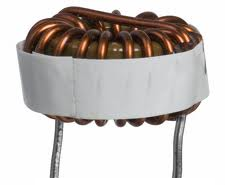﻿ Inductor Characteristics/Specifications- Explained ﻿# Inductor Characteristics/Specifications- ExplainedThere are many characteristics and specifications which appear on an inductor's datasheet which holds significant value to the nature of the inductor.

These include terms such as the curie temperature, the inductor's DC resistance (DCR), electromagnetic interference, and magnetic saturation flux density.

What do all of these terms mean? Well, in this article, we will go over each practically so that you can understand what each specification is and how it determines whether the inductor can suit and function appropriately in a particular circuit.

### DC Resistance (DCR)

The DC Resistance (DCR) specification of an inductor is the amount of resistance that an inductor has for signals with frequencies of or near 0Hz.

Inductors are devices that allow DC signals to pass through without almost any impediment, but which block the passing of high-frequency signals. Inductors have very low resistance for low-frequency signals, while displaying very high resistance for signals with high frequencies.

The DCR of an inductor is normally very small, ranging from less than 1/100 of 1Ω to a few ohms (usually no greater than 4Ω). The smaller inductor values normally have a lower DCR, while the larger inductor values have a higher one.

The DCR of an inductor is measured using DC current. DC current is fed into the inductor and then the resistance can be calculated from the voltage and current in the circuit using R=V/I.

The DCR is meant to be minimized in the design of an inductor, meaning it is designed to be kept low. Inductors are not made to block DC signals, so they should have a low DCR. The DCR value in a datasheet is specified as a maximum rating, meaning the value specified in the datasheet is the maximum resistance that it will contain. The DC resistance of the inductor should not exceed the DCR specified.

### Maximum DC Current

The Maximum DC Current specification of an inductor is the maximum level of continuous direct current that can be passed through an inductor with no damage.

### Electromagnetic Interference

The Electromagnetic Interference, for inductors, refers to the amount of magnetic field radiated away from the inductor into space. The field may cause interference with other magnetically sensitive components and may require consideration in circuit design and layout.

### Magnetic Saturation Flux Density

The Magnetic Saturation Flux Density (Bsat) specification of an inductor is the inductor parameter that indicates the maximum flux the core material can be induced to hold. At this value of flux density, all magnetic domains within the core are magnetized and aligned.

### Curie Temperature

Curie Temperature (TC) is the temperature beyond which the core material of the inductor loses its magnetic properties.

Related Resources